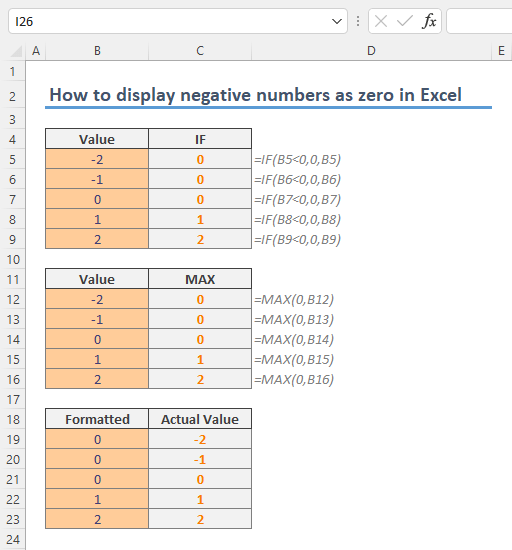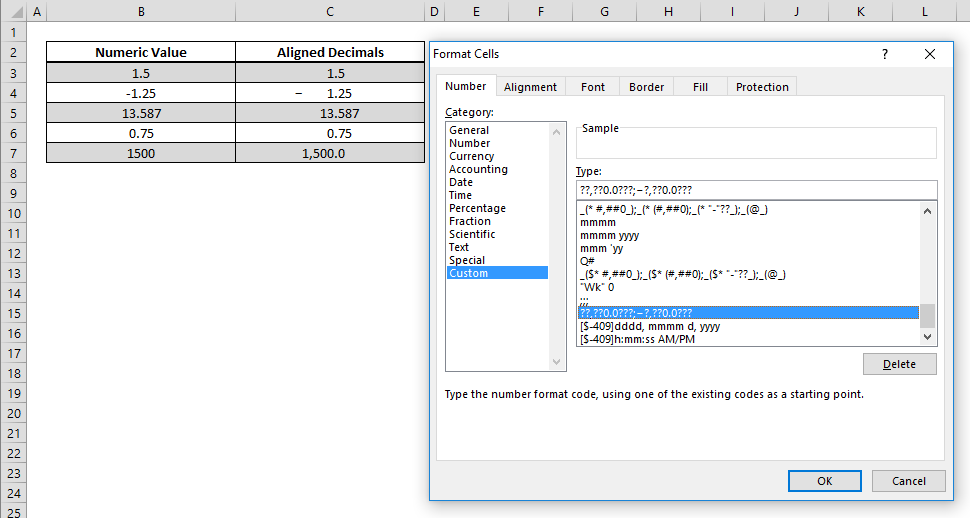## How to display negative numbers as zero in Excel

In this guide, we’re going to show you How to display negative numbers as zero in Excel in two ways. Download-Workbook Formula approach to display negative numbers as zero The first method in our list is the formula way. An obvious way is to use the IF function which...## How to display fractions in Excel

Excel usually converts fractions automatically when you enter a date or text value. In this guide, we’re going to show you how to display fractions in Excel. Download Workbook Using number formatting to display fractions Excel stores and calculates any numerical value...## How to calculate percentage in Excel

A percentage is a number or ratio that represents a fraction of 100, and this representation is used widely from interest rates to risk analysis, or really in any application when the contribution of a specific item to the whole is to be highlighted. In this article,...## How to line up decimals in Excel using Number Formatting

Lining up the decimal places is the desired structure for some applications, especially in a finance context. With this, you can make the decimal portion of numbers start from the same place, regardless of how many digits the consist of. You can do this using...## How to create a Quarter formula in Excel

There is no specific Quarter formula in Excel. But an Excel quarter formula can be constructed by combining ROUNDUP and MONTH functions with custom number formatting. Syntax =ROUNDUP( MONTH( date value ) /3, 0 ) Steps Start with =ROUNDUP( function Continue with MONTH(...